15/05/2019

## I have used STATA/SPSS for years, why learn R?

Because:

• R is free
• R is open source and has excellent package ecosystem
• R is very versatile
• R is very good for data visualisation
• R can accomodate more than one data frame in memory at the same time

On the other hand:

• R has a steep(er) learning curve

## Popularity of data analysis software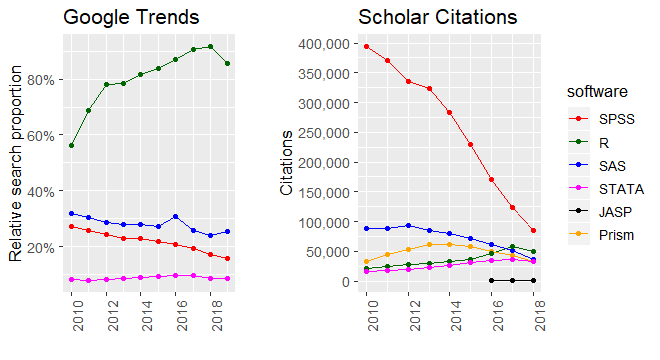More discussion on this can be found here.

## R and RStudio

• As in STATA, you can either use a script (do file) to save commands and then run them, or write commands directly into the console
• It is perfectly possible to use the base R environment for everything, but most people do not do this
• Today we will mostly be using RStudio, which is a graphical user interface (GUI) for R
• RStudio has a host of useful features which make it an excellent environment for learning R
• Intuitive interface
• Syntax highlighting
• Easy data overview
• Project management

## Basic mathematical operations in R

`1 + 1`
`##  2`
`5 - 3`
`##  2`
`6 / 2`
`##  3`
`4 * 4`
`##  16`

## Basic mathematical operations in R

• Other typical mathematical functions are also hard-coded:
```sum(<numbers>) # Sum
mean(<numbers>) # Mean
median(<numbers>) # Median
sd(<numbers>) # Standard Deviation
log(<number>) # Logarithm
exp(<number>) # Exponent
sqrt(<number>) # Square root```

## Basic operations in R

R also understands logical operators:

• `<` : less than
• `>` : greater than
• `==` : equal to (note, not `=`)
• `>=` : greater than or equal to
• `<=` : less than or equal to
• `!=` : not equal to
• `&` : and
• `|` : or

## R `objects`

• The entities that R creates and manipulates are known as objects
• These may be vectors, matrices, character strings, functions
• Objects are created using the assignment operator: `<-`
• Once created, an object is stored in your current global environment
```x1 <- 10
print(x1)```
`##  10`
```x2 <- 4
x3 <- x1 - x2
print(x3)```
`##  6`

## `vector` - the basic building block of R.

A vector is a collection of elements which all share the same type.

We use the `c()` function to concatenate observations into a single vector.

• Numeric
```num_vec <- c(150, 178, 67.7, 905, 12)
print(num_vec)```
`##  150.0 178.0  67.7 905.0  12.0`
`num_vec`
`##  150.0 178.0  67.7 905.0  12.0`

## `vector` - the basic building block of R.

There are no scalars (atomic units) in R

A scalar is just a vector of length 1

• Character
```char_vec <- c("apple", "pear", "plum", "pineapple", "strawberry")
length(char_vec)```
`##  5`
```char_scalar <- "banana"
length(char_scalar)```
`##  1`

## `vector` - the basic building block of R.

When creating new objects, keep in mind reserved words and try to avoid using them in names

• Logical (Boolean)
```log_vec <- c(FALSE, FALSE, FALSE, TRUE, TRUE)
log_vec```
`##  FALSE FALSE FALSE  TRUE  TRUE`
```log_vec2 <- c(F, F, F, T, T)
log_vec2```
`##  FALSE FALSE FALSE  TRUE  TRUE`

## `vector` - the basic building block of R.

• Factor

A factor is similar to a character vector, but here each unique element is associated with a numerical value which represents a category:

```fac_vec <- as.factor(c("a", "b", "c", "b", "b", "c"))
fac_vec```
```##  a b c b b c
## Levels: a b c```
`as.numeric(fac_vec)`
`##  1 2 3 2 2 3`

## `vector` - the basic building block of R.

• Date

A date is similar to a numeric vector, as it stores the number of days, but it can assume a variety of forms:

```date_vec <- as.Date(c("2001/07/06", "2005/05/05", "2010/06/05", "2015/07/05", "2017/08/06"))
date_vec```
`##  "2001-07-06" "2005-05-05" "2010-06-05" "2015-07-05" "2017-08-06"`
`as.numeric(date_vec)`
`##  11509 12908 14765 16621 17384`
`format(date_vec, "%d/%m/%Y")`
`##  "06/07/2001" "05/05/2005" "05/06/2010" "05/07/2015" "06/08/2017"`

## `vector` subsetting

To subset a `vector`, use square parenthesis to index the elements you would like via `object[index]`:

• Numerical subsetting
`char_vec`
`##  "apple"      "pear"       "plum"       "pineapple"  "strawberry"`
`char_vec[1:3]`
`##  "apple" "pear"  "plum"`

## `vector` subsetting

Instead of passing indices, it is also possible to use a logical vector to specify the required elements:

• Logical subsetting
`log_vec`
`##  FALSE FALSE FALSE  TRUE  TRUE`
`char_vec[log_vec]`
`##  "pineapple"  "strawberry"`

## `vector` operations

In R, mathematical operations on vectors occur elementwise:

```fib <- c(1, 1, 2, 3, 5, 8, 13, 21)
fib[1:7]```
`##   1  1  2  3  5  8 13`
`fib[2:8]`
`##   1  2  3  5  8 13 21`
`fib[1:7] + fib[2:8]`
`##   2  3  5  8 13 21 34`

## `vector` operations

It is also possible to perform logical operations on vectors:

```fib <- c(1, 1, 2, 3, 5, 8, 13, 21)
fib_gt_5 <- fib > 5
fib_gt_5```
`##  FALSE FALSE FALSE FALSE FALSE  TRUE  TRUE  TRUE`

We can also combine logical operators

```fib_gt_5_or_ls_2 <- fib > 5 | fib < 2
fib_gt_5_or_ls_2```
`##   TRUE  TRUE FALSE FALSE FALSE  TRUE  TRUE  TRUE`

## `matrix`

A matrix is just a collection of vectors! I.e. it is a 2-dimensional vector that has 2 additional attributes: the number of rows and columns

As for vectors all elements of a matrix must be of the same type

```mat <- matrix(data = 1:25, nrow = 5, ncol = 5)
mat```
```##      [,1] [,2] [,3] [,4] [,5]
## [1,]    1    6   11   16   21
## [2,]    2    7   12   17   22
## [3,]    3    8   13   18   23
## [4,]    4    9   14   19   24
## [5,]    5   10   15   20   25```

## `list`

A `list` is a collection of R objects of different types

```l <- list(element1 = num_vec,
element2 = mat[1:2,1:2],
element3 = "Farmers' Market")
l```
```## \$element1
##  150.0 178.0  67.7 905.0  12.0
##
## \$element2
##      [,1] [,2]
## [1,]    1    6
## [2,]    2    7
##
## \$element3
##  "Farmers' Market"```

## `list` subsetting

Individual elements of a `list` object can be subset using `\$` or `[[` operators:

`l\$element2`
```##      [,1] [,2]
## [1,]    1    6
## [2,]    2    7```
`l[]`
```##      [,1] [,2]
## [1,]    1    6
## [2,]    2    7```

## `data.frame` - the workhorse of data analysis.

A `data.frame` is an R object in which the columns can be of different types

Despite their matrix-like appearance, data frames in R are lists, where all elements are of equal length!

```df <- data.frame(weight = num_vec, fruit = char_vec, berry = log_vec)
df```
```##   weight      fruit berry
## 1  150.0      apple FALSE
## 2  178.0       pear FALSE
## 3   67.7       plum FALSE
## 4  905.0  pineapple  TRUE
## 5   12.0 strawberry  TRUE```

## `matrix` and `data.frame` subsetting

To subset a `matrix` or `data.frame`, you need to specify both rows and columns:

`mat[1:3, 1:3]`
```##      [,1] [,2] [,3]
## [1,]    1    6   11
## [2,]    2    7   12
## [3,]    3    8   13```
`df[1, ]`
```##   weight fruit berry
## 1    150 apple FALSE```

## `matrix` and `data.frame` subsetting

We can also subset to remove rows or columns by using the `-` operator applied to the `c` function:

`mat[-c(1:3), -c(1:3)]`
```##      [,1] [,2]
## [1,]   19   24
## [2,]   20   25```

In the code, `1:3` creates a vector of the integers 1, 2 and 3, and the `-` operator negates these. We wrap the vector in the `c` function so that `-` applies to each element, and not just the first element.

## `functions` - the backbone of R operations.

All operations on objects in R are done with functions.

`fun(arg1, arg2, ...)`

Where

• `fun` is the name of the function
• `arg1` is the first argument passed to the function
• `arg2` is the second argument passed to the function

## `function` example

Let's consider the `mean()` function. This function takes two main arguments:

`mean(x, na.rm = FALSE)`

Where `x` is a numeric vector, and `na.rm` is a logical value that indicates whether we'd like to remove missing values (`NA`).

```vec <- c(1, 2, 3, 4, 5)
mean(x = vec, na.rm = FALSE)```
`##  3`
```vec <- c(1, 2, 3, NA, 5)
mean(x = vec, na.rm = TRUE)```
`##  2.75`

## `function` example

We can also perform calculations on the output of a function:

`mean(num_vec) * 3`
`##  787.62`

Which means that we can also have nested functions:

`log(mean(num_vec))`
`##  5.570403`

We can also assign the output of any function to a new object for use later:

`log_fruit <- log(mean(num_vec)) # The logarithm of average fruit weight`

## Basic operations are `functions` in disguise!

All the basic operations on R `objects` that we have encountered so far are, in fact, `functions`:

``+`(1,1)`
`##  2`
``-`(5,3)`
`##  2`
``[`(char_vec, 1:3)`
`##  "apple" "pear"  "plum"`

## User defined `functions`

Functions are also objects, and we can create our own. We define a function as follows:

```sum2 <- function(a, b){
return(a + b)
}

res <- sum2(a = 5, b = 50)
res```
`##  55`

Note that the function itself, and the result returned by the function are both objects!

## Help!

```?summary
help(summary)```

The quality of the help varies hugely across packages.

Stackoverflow is a good resource for many standard tasks.

For custom packages it is often helpful to check the issues page on the GitHub.

E.g. for `ggplot2`: https://github.com/tidyverse/ggplot2/issues

Or, indeed, any search engine #LMDDGTFY.

## Reading data into R (.csv)

`sim.df <- read.csv(file = "file.csv")`
• `df` is an R data.frame object (you could call this anything)
• `file.csv` is a .csv file with your data
• `<-` is the assignment operator
• In order for R to access `file.csv`, it will have to be saved in your current working directory
• Before recently, the standard advice was to use `getwd()` and `setwd()`.
• Try to avoid it! Instead, create new RStudio project and use relative paths:
`sim.df <- read.csv(file = "./data/file.csv")`
• More details can be found here

## Reading data into R (STATA .dta, SPSS .sav)

```install.packages("haven") # Install the "haven" package
sim.df <- haven::read_sav(file = "./data/file.sav") ```
• The `haven` package is not automatically installed in R so we need to do that ourselves
• `df` is still a R data.frame objects
• `file.dta` is a .dta (used by STATA) file with your data
• `file.sav` is a .sav (used by SPSS) file with your data
• `<-` is the usual assignment operator

## Descriptive statistics `functions`

```View(sim.df) # View data in spreadsheet-style
names(sim.df) # Names of the variables in your data
head(sim.df) # First n (six, by default) rows of the data
tail(sim.df) # Last n (six, by default) rows of the data
str(sim.df) # "Structure" of any R object
summary(sim.df) # Summary statistics for each of the columns in the data
dim(sim.df) # Dimensions of data
mean(sim.df\$var) # Mean of a numeric vector
sd(sim.df\$var) # Standard deviation of a numeric vector
range(sim.df\$var) # Range of a numeric vector
quantile(sim.df\$var) # Quantiles of a numeric vector```

## `str`

`str()` applied to data frames is particularly useful in inferring types of variables in the data

`str(sim.df) `
```## 'data.frame':    1000 obs. of  4 variables:
##  \$ x: num  1.807 -1.249 -0.136 0.951 -1.271 ...
##  \$ y: num  1.134 0.596 0.8 2.265 0.889 ...
##  \$ z: num  0.0869 0.2752 0.7046 0.6106 0.8831 ...
##  \$ g: Factor w/ 6 levels "a","b","c","d",..: 4 5 1 1 3 6 1 3 6 5 ...```

## `summary`

`summary(sim.df) `
```##        x                    y                 z             g
##  Min.   :-2.7467901   Min.   :-3.2166   Min.   :0.0007827   a:177
##  1st Qu.:-0.6505946   1st Qu.:-0.2343   1st Qu.:0.2568557   b:161
##  Median : 0.0003546   Median : 0.4703   Median :0.4932571   c:173
##  Mean   : 0.0161324   Mean   : 0.4774   Mean   :0.4954730   d:141
##  3rd Qu.: 0.6626705   3rd Qu.: 1.1694   3rd Qu.:0.7341048   e:177
##  Max.   : 3.0778088   Max.   : 3.5594   Max.   :0.9994499   f:171```

## `pairs`

`pairs()` produces a matrix of bivariate scatterplots

`pairs(sim.df)`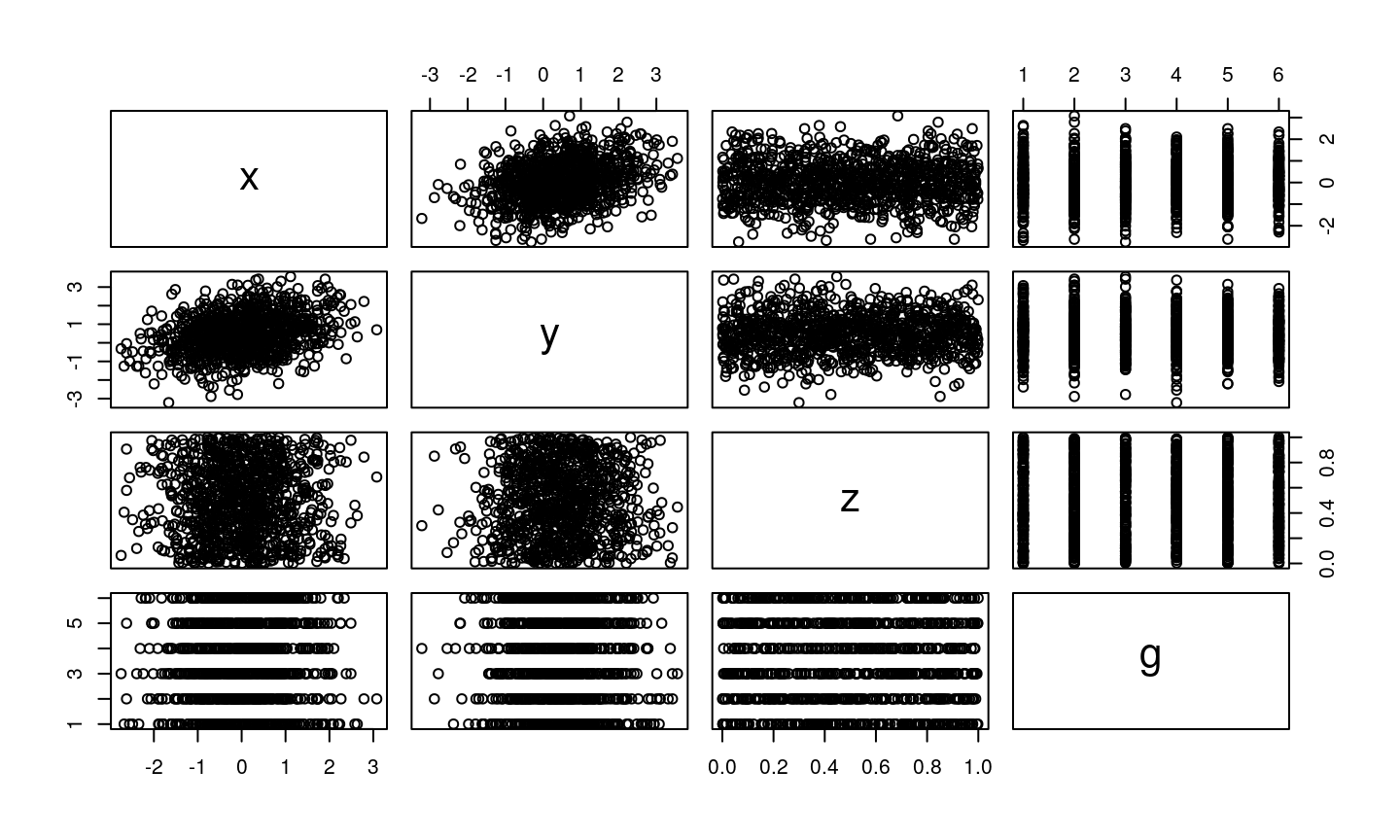## `range`

Suppose you would like to find the range of a variable in your `data.frame`. The following will not work:

`range(sim.df) `
`## Error in FUN(X[[i]], ...): only defined on a data frame with all numeric variables`

Right, we cannot take the range of the entire data! Some of the variables are not numeric, and therefore do not have a range…

## `range`

Instead, we need to access one variable. There are two ways to do this.

• We can subset using square parenthesis, or
• We can use the `[[` operator and access the variable of interest by position, or
• We can use the `\$` operator and access the variable of interest by name

Note that the last two treat `data.frame` as a list with variables as elements

```range(sim.df[,1])
range(sim.df[])```
`range(sim.df\$x) `
`##  -2.746790  3.077809`

## Introduction

• Plots are one of the great strenghts of R
• There are two main frameworks for plotting
• Base R graphics
• `ggplot`
• Which should you use? It is a matter of preference!

## Base R plots

The basic plotting syntax is very simple. `plot(x_var, y_var)` will give you a scatter plot:

`plot(sim.df\$x, sim.df\$y)`

## Base R plots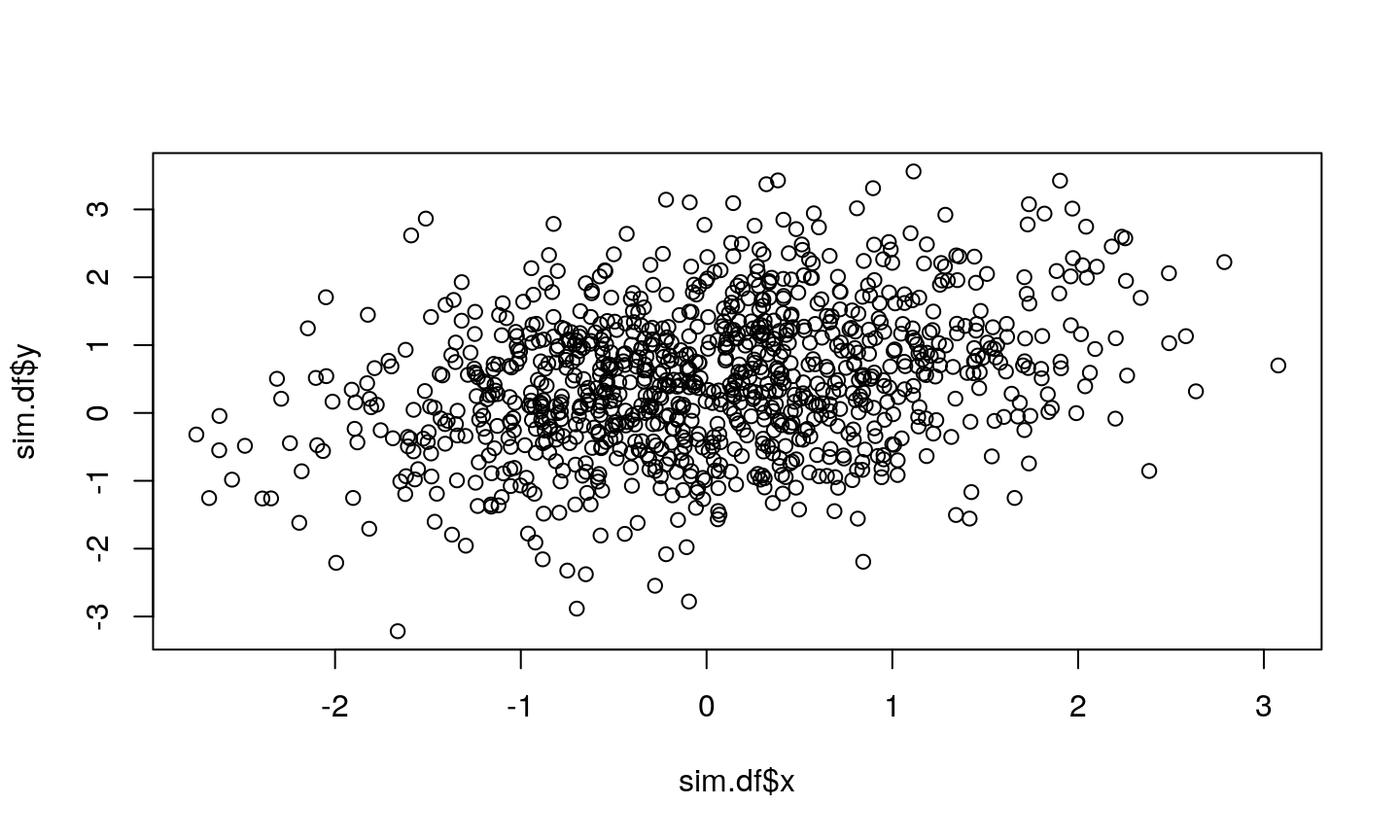Hmm, let's work on that.

## Base R plots

The plot function takes a number of arguments (`?plot` for a full list). The fewer you specify, the uglier your plot:

```plot(x = sim.df\$x, y = sim.df\$y,
xlab = "X variable",
ylab = "Y variable",
main = "Awesome plot title",
pch = 19, # Solid points
cex = 0.5, # Smaller points
bty = "n", # Remove surrounding box
col = sim.df\$g # Colour by grouping variable
)```

## Base R plots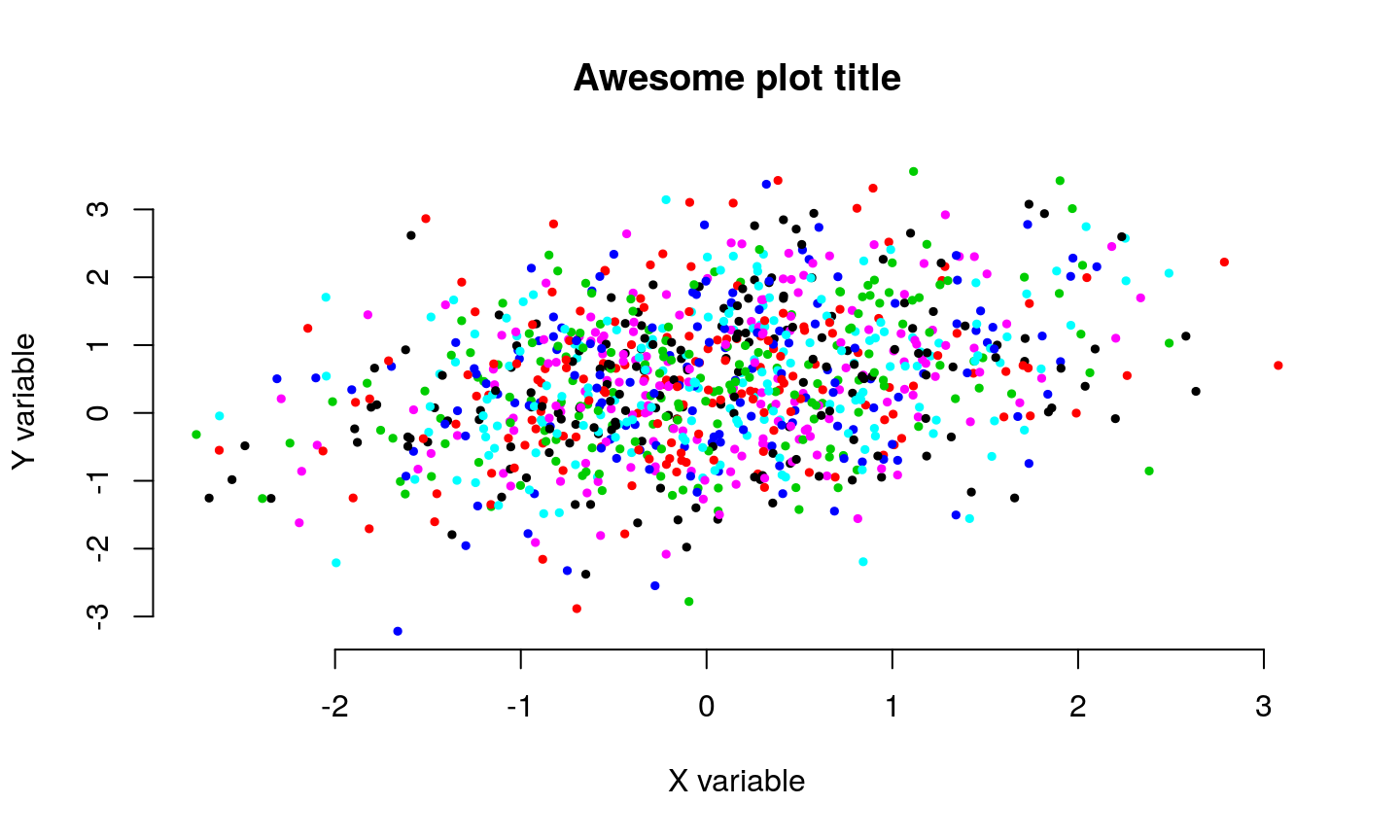## Base R plots

The default behaviour of `plot()` depends on the type of input variables for the `x` and `y` arguments. If `x` is a factor variable, and `y` is numeric, then R will produce a boxplot:

`plot(x = sim.df\$g, y = sim.df\$x)`

## Base R plots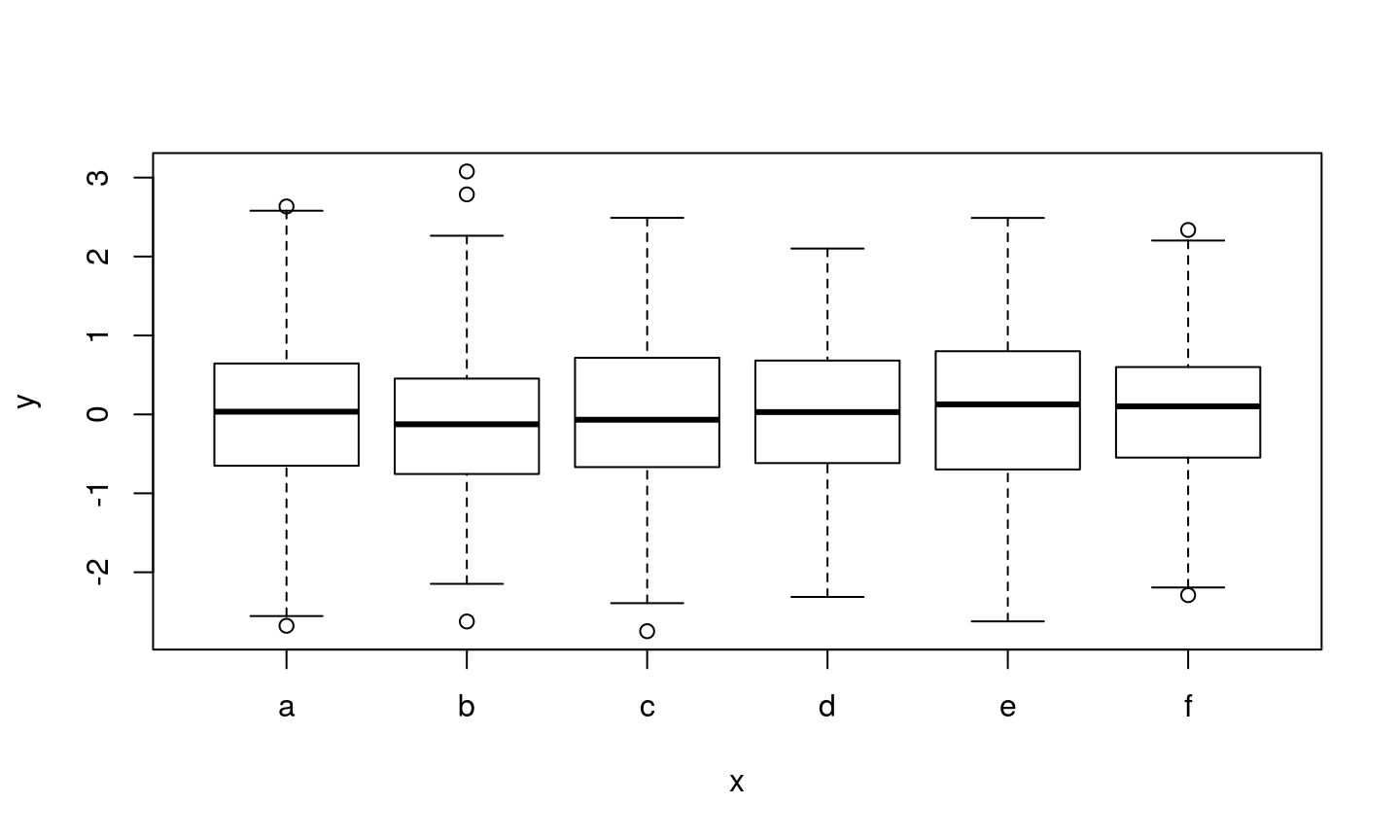## `ggplot`

A very popular alternative to base R plots is the `ggplot2` library (the 2 in the name refers to the second iteration, which is the standard). This is a separate package (i.e. it is not a part of the base R environment) but is very widely used.

• Based on the Grammar of Graphics data visualisation scheme:

Wilkinson, L. (2005). The Grammar of Graphics 2nd Ed. Heidelberg: Springer. https://doi.org/10.1007/0-387-28695-0

Wickham, H. (2010). A Layered Grammar of Graphics. Journal of Computational and Graphical Statistics, 19(1), 3–28. https://doi.org/10.1198/jcgs.2009.07098

• Graphs are broken into multiple layers

• Layers can be recycled across multiple plots

## `ggplot`

Let's recreate the previous scatter plot using `ggplot`:

```library("ggplot2")
ggplot(data = sim.df, aes(x = x, y = y, col = g)) +